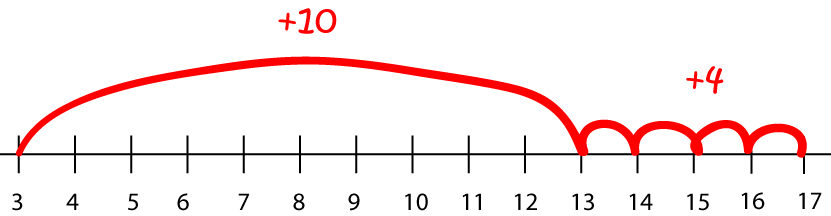Pull Down & Release to Refresh

# Definition of Jump StrategyThe jump strategy is a strategy in which a calculation is completed by jumping by easy parts of the number being added or subtracted.

For example, we could find $3 + 14$ by calculating $3 + 14 = 3 + 10 + 1 + 1 + 1 + 1 = 17$.

We could find $23 - 14$ by calculating $23 - 14 = 23 - 13 - 1 = 9.$

### Description

The aim of this dictionary is to provide definitions to common mathematical terms. Students learn a new math skill every week at school, sometimes just before they start a new skill, if they want to look at what a specific term means, this is where this dictionary will become handy and a go-to guide for a student.

### Audience

Year 1 to Year 12 students

### Learning Objectives

Learn common math terms starting with letter J

Author: Subject Coach
You must be logged in as Student to ask a Question.